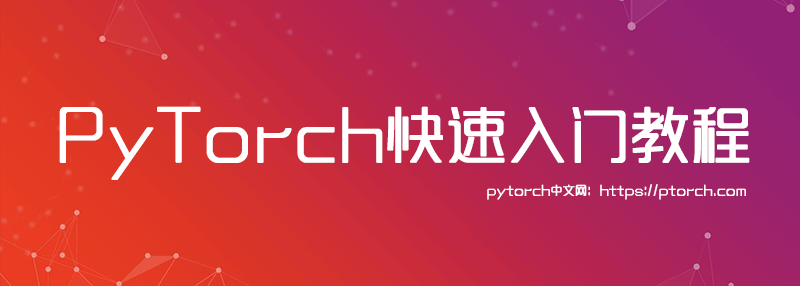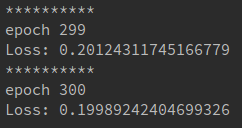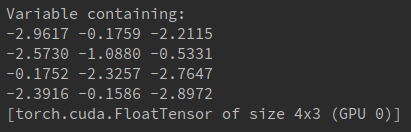PyTorch快速入门教程九（使用LSTM来做判别每个词的词性） 顶转LSTM 词性判断

LSTM的网络结构在之前已经介绍过了.首先，我们定义好一个LSTM网络，然后给出一个句子，每个句子都有很多个词构成，每个词可以用一个词向量表示，这样一句话就可以形成一个序列，我们将这个序列依次传入LSTM，然后就可以得到与序列等长的输出，每个输出都表示的是一种词性，比如名词，动词之类的，还是一种分类问题，每个单词都属于几种词性中的一种。

准备数据

training_data = [
("The dog ate the apple".split(), ["DET", "NN", "V", "DET", "NN"]),
("Everybody read that book".split(), ["NN", "V", "DET", "NN"])
]

word_to_idx = {}
tag_to_idx = {}
for context, tag in training_data:
for word in context:
if word not in word_to_idx:
word_to_idx[word] = len(word_to_idx)
for label in tag:
if label not in tag_to_idx:
tag_to_idx[label] = len(tag_to_idx)

alphabet = 'abcdefghijklmnopqrstuvwxyz'
character_to_idx = {}
for i in range(len(alphabet)):
character_to_idx[alphabet[i]] = i

字符LSTM

class CharLSTM(nn.Module):
def __init__(self, n_char, char_dim, char_hidden):
super(CharLSTM, self).__init__()
self.char_embedding = nn.Embedding(n_char, char_dim)
self.char_lstm = nn.LSTM(char_dim, char_hidden, batch_first=True)

def forward(self, x):
x = self.char_embedding(x)
_, h = self.char_lstm(x)
return h

词性LSTM

class LSTMTagger(nn.Module):
def __init__(self, n_word, n_char, char_dim, n_dim, char_hidden,
n_hidden, n_tag):
super(LSTMTagger, self).__init__()
self.word_embedding = nn.Embedding(n_word, n_dim)
self.char_lstm = CharLSTM(n_char, char_dim, char_hidden)
self.lstm = nn.LSTM(n_dim+char_hidden, n_hidden, batch_first=True)
self.linear1 = nn.Linear(n_hidden, n_tag)

def forward(self, x, word_data):
word = [i for i in word_data]
char = torch.FloatTensor()
for each in word:
word_list = []
for letter in each:
word_list.append(character_to_idx[letter.lower()])
word_list = torch.LongTensor(word_list)
word_list = word_list.unsqueeze(0)
tempchar = self.char_lstm(Variable(word_list).cuda())
tempchar = tempchar.squeeze(0)
char = torch.cat((char, tempchar.cpu().data), 0)
char = char.squeeze(1)
char = Variable(char).cuda()
x = self.word_embedding(x)
x = torch.cat((x, char), 1)
x = x.unsqueeze(0)
x, _ = self.lstm(x)
x = x.squeeze(0)
x = self.linear1(x)
y = F.log_softmax(x)
return y

运行结果Pytorch中文文档

Pytorch中文论坛

Pytorch中文文档已经发布，完美翻译，更加方便大家浏览：

Pytorch中文网：https://ptorch.com/

Pytorch中文文档：https://ptorch.com/docs/1/

2018/07/12
0
0

2018/10/22
0
0
PyTorch 你想知道的都在这里

2018/10/20
0
0
PyTorch 官方中文教程包含 60 分钟快速入门教程，强化教程

PyTorch 是一个基于 Torch 的 Python 开源机器学习库，用于自然语言处理等应用程序。它主要由 d 的人工智能小组开发，不仅能够 实现强大的 GPU 加速，同时还支持动态神经网络，这一点是现在很...

08/14
0
0
PyTorch 高级实战教程：基于 BI-LSTM CRF 实现命名实体识别和中文分词

04/12
0
0

Spring Boot 2 实战：使用 Spring Boot Admin 监控你的应用

1. 前言 生产上对 Web 应用 的监控是十分必要的。我们可以近乎实时来对应用的健康、性能等其他指标进行监控来及时应对一些突发情况。避免一些故障的发生。对于 Spring Boot 应用来说我们可以...

6
0
ZetCode 教程翻译计划正式启动 | ApacheCN

ApacheCN_飞龙

4
0
CSS定位

CSS定位 relative相对定位 absolute绝对定位 fixed和sticky及zIndex relative相对定位 position特性：css position属性用于指定一个元素在文档中的定位方式。top、right、bottom、left属性则...

studywin

7
0

Java技术江湖

7
0

Moks角木

7
0